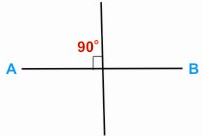HOME MATH DICTIONARY DOWNLOAD FEEDBACK DISCLAIMER
 Question: What is Right Angle ? Answer: A right angle is the name given to a quarter of a turn and is equal to 90o. It is the angle between vertical and horizontal, the angle between north and west. In geometry and trigonometry, a right angle is an angle of 90 degrees, corresponding to a quarter turn (that is, a quarter of a full circle). It can be defined as the angle such that twice that angle amounts to a half turn, or 180o. Many instruments have been designed to draw a right angle, such as a set square and T-square, because of its importance in construction and building. In mathematical drawings, a right angle is indicated by a small box. Lines that are at a right angle to each other are perpendicular, an important geometrical property. The presence of a right angle in a triangle is the defining factor for right triangles. The word angle comes from the Latin word angulus, meaning a corner. The word angulus is a diminutive, of which the primitive form, angus, does not occur in Latin.Example of a Right Angle: